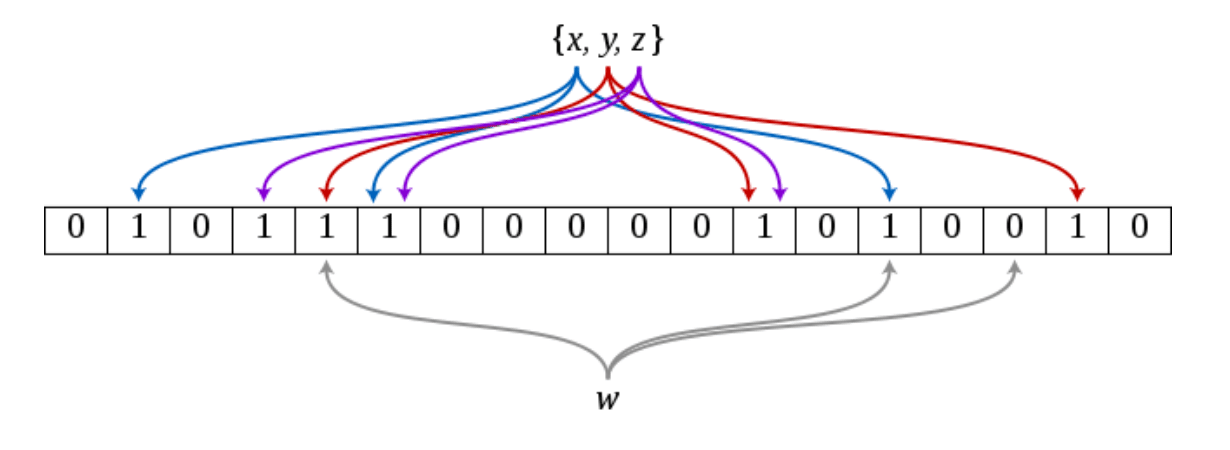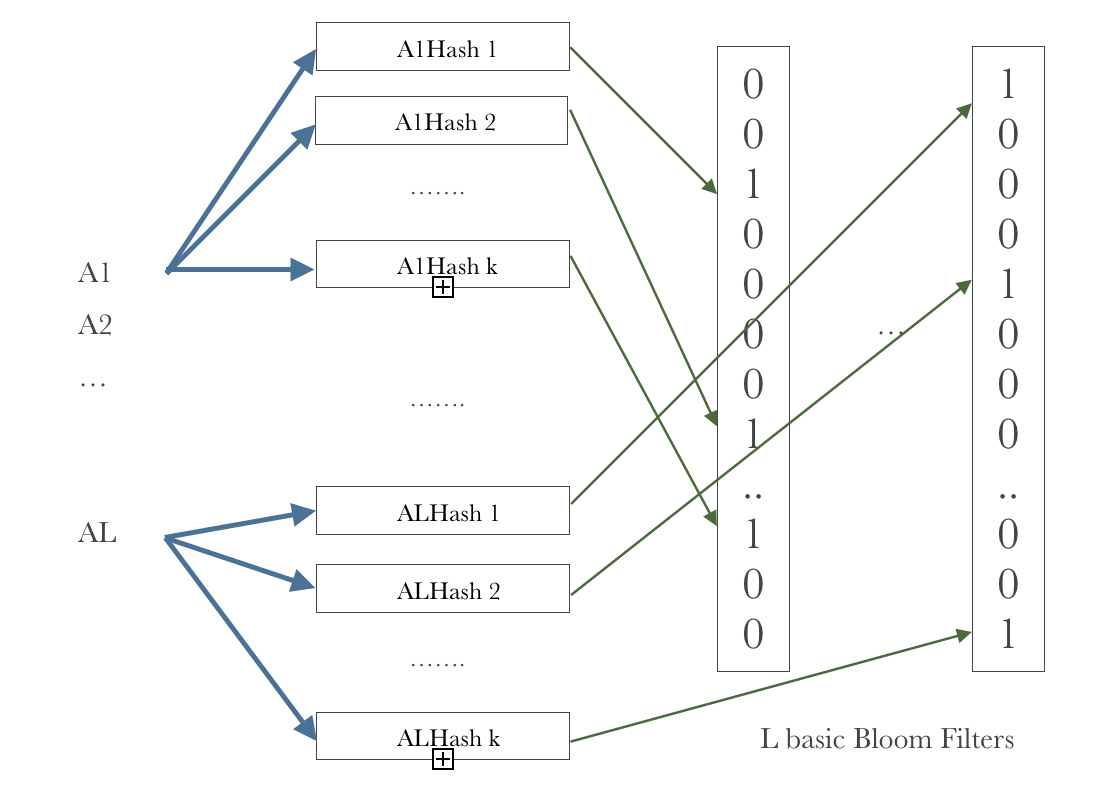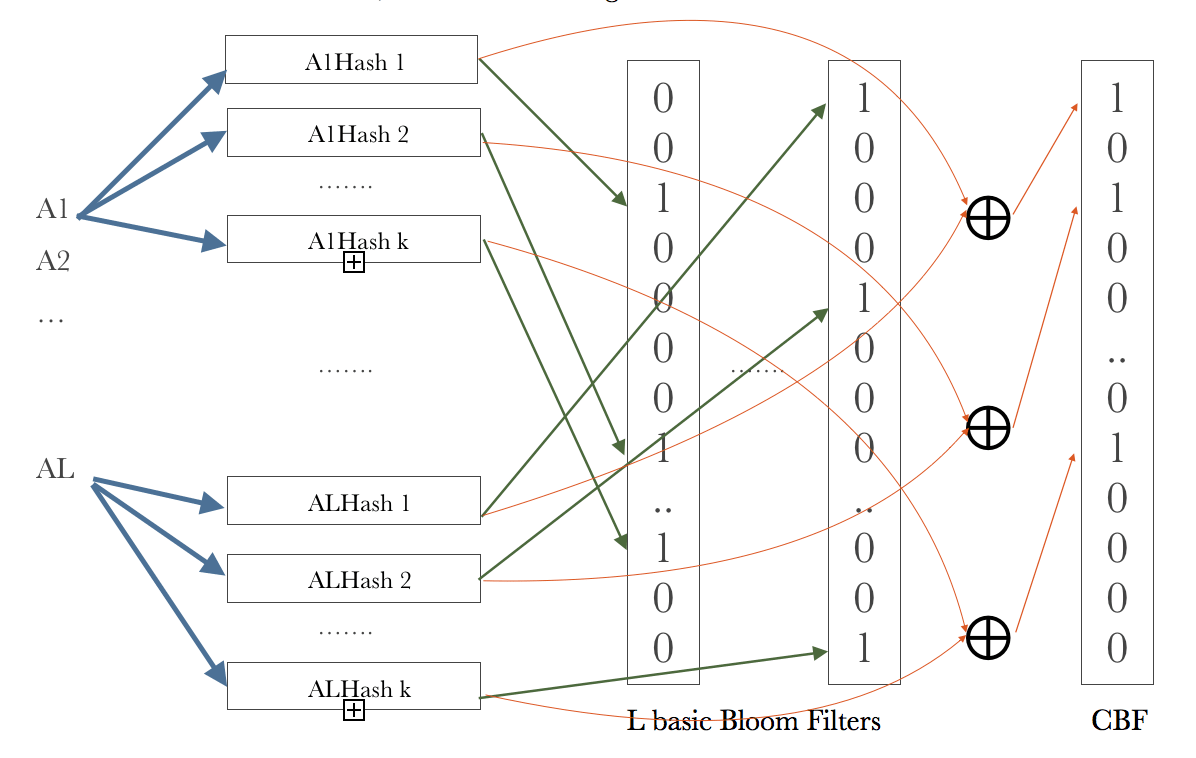# NIUHE

### Combined Multi-dimension Bloom Filter

​ A Bloom filter is a space-efficient probabilistic data structure, conceived by Burton Howard Bloom in 1970, that is used to test whether an element is a member of a set. False positive matches are possible, but false negatives are not – in other words, a query returns either "possibly in set" or "definitely not in set". Elements can be added to the set, but not removed (though this can be addressed with a "counting" filter); the more elements that are added to the set, the larger the probability of false positives.

## Standard Bloom Filter

​ Let’s briefly review the standard bloom filter. A bloom filter is used to represent a set $S = \{s_1, s_2, ...,s_n\}$ with a m-bit array and each bit of which is denoted by $BF, BF, …, BF[m-1]$. Initially, the m-bit array is initiated with 0 and we define hash functions. Each of them can reflect each element $x \in S$ to a number $h_i(x) \in \{0, 1, ..., m-1\}$ randomly, where $i \in \{0, 1, .., k-1\}$. Whenever inserting an element, hashing it with the $k$ hash functions, so we get $k$ indexes. Then letting $BF[h_i(x)] = 1$ for each $i \in \{0, 1, .., k-1\}$. Given an element $y$, we check each $BF[h_i(y)]$ , if all of them equal to $1$ , then we assume is in the set $S$ even though it may actually not. Hence a Bloom Filter may yield false positives. However, if there is one of them equals to $0$, then the element $y$ is definitely not in the set $S$ .

​ For example, after inserting $x,y,z$ , if we want to figure out whether $w$ is in the set, since $BF[h_2(w)] = 0$ , the Bloom Filter will tell us is not in the set, as illustrated in the figure Figure 1.Figure 1 An example of Bloom Filter

​ In order to analyze the possibility that a Bloom Filter gives a false positive, we denote a Bloom Filter with a three-element tuple $(n,m,k)$ and further assume that each $\{0, 1, ..., m-1\}$ hash function is uniform distributed, which means the number in has equal possibility to be chosen by a hash function. Therefore, given a hash function, the possibility of one specific position in BF equals to 0 is: $1 - \frac{1}{m}$ Then, with respect to all hash functions, the possibility becomes to: $(1-\frac{1}{m})^k$ After inserted elements, the possibility is: $(1-\frac{1}{m})^{nk}$ If a specific item leads the Bloom Filter to yield a false positive, all of the results of must equal to 1, whose possibility is: $[1-(1-\frac{1}{m})^{nk}]^k$ According to the definition of , we can approximately represent the possibility as: $f_{BBF} = [1-(1+\frac{1}{(-m)})^{(-m)\frac{nk}{(-m)}}]^k \approx (1-e^{-\frac{kn}{m}})^k$ The standard Bloom Filter is basic for extension of Bloom Filters. From now on, it is termed basic Bloom Filter (BBF).

## Multi-dimension Bloom Filter

​ In order to store multi-dimension data, for example $l$ dimensions, we can simply use $l$ basic Bloom Filters to store each dimension, which called a Multi-dimension Bloom Filter (MDBF). When we want to identify whether element $x$ is in the set, just compute $BBF_1[x_0], ..., BBF_l[x_{l-1}]$, if all of them equal to $1$, then we consider $x$ is one of the elements of the set. Similarly as the basic Bloom Filter, such multi-dimension Bloom Filter may also yield a false positive. However, if one of them equals to $0$, the element $x$ certainly not belong to this set. Figure 2 illustrates that how a MDBF works.Figure 2 Multi-dimension Bloom Filter illustration

​ Nonetheless, the false positive rate of such MDBF is not as ideal as expected, because it may give a false positive even if none of the basic Bloom Filters makes a false decision. For example, we have a set $S = \{(red, blue), (blue, black)\}$ and we want to figure out whether $(red, black)$ is in $S$. So the first BBF would say that is an attribute of the set and the second BBF says $black$ is also an attribute of $S$, so the MDBF thinks $(red, black)$ is a member of $S$, which is obviously a false positive. Therefore, we need another Bloom Filter or couple of Bloom Filters to represent the combined information of each dimensional attribute to reduce the error rate.

## Combined Multi-dimension Bloom Filter

​ The ideal MDBF produces a false positive only when all of the basic Bloom Filters yield false positives, so the error rate of an ideal -dimension MDBF is: $f_{iMDBF} = (f_{BBF})^l = (1-e^{-\frac{kn}{m}})^{kl}$ ​ To achieve such false positive rate, we need not only store the attribute of each dimension, but also have to store the relationship among all dimensions. One way to do this is adding a combined Bloom Filter to represent the combination of each attribute, which is called combined multi-dimension Bloom Filter (CMDBF). The question is how to represent such combined information. A simple solution is using the result of XOR different dimensional attributes’ hashing indexes as the hash index of the combined Bloom Filter, which is $CBF[h_{1,i}[x_0]\oplus h_{2,i}[x_1] ... \oplus h_{l,i}[x_{l-1}]]=1$ for $i \in \{0, 1, ..,k-1\}$, where $CBF$ refers to the combined Bloom Filter, as illustrated in Figure 3.Figure 3 Combined Multi-dimension Bloom Filter illustration

​ Obviously, the error rate of CMDBF is lower than MDBF since CMDBF has a combined Bloom Filter to represent the relationship among all the dimensions. CMDBF will make a false positive when some of the basic Bloom Filters make mistakes as well as the combined Bloom Filter yields a false decision.

​ Let’s mathematically compute the error rate of CMDBF, we represent a CMDBF by a 4-tuple $(n,m,k,l)$, where $n$ represents the number of elements in the set, $m$ represents the size of a basic Bloom Filter, $k$ represents the number of hash functions each basic Bloom Filter has, and $l$ represents the dimensions of the elements. Since $h_{1,i}[x_0], h_{2,i}[x_1] ... , h_{l,i}[x_{l-1}] \sim U(0, m-1)$ where , therefore, $h_{1,i}[x_0]\oplus h_{2,i}[x_1] ... \oplus h_{l,i}[x_{l-1}]\sim U(0,m-1)$ So, the false positive rate of CBF equals to that of BBF, $f_{CBF} = f_{BBF} = (1-e^{-\frac{kn}{m}})^k$ Then, the error rate of CMDBF is $f_{CMDBF} = f_{MDBF}f_{CBF} = f_{MDBF}(1-e^{-\frac{kn}{m}})^k$ Since $0<(1-e^{-\frac{kn}{m}})^k<1$, the false positive rate of CMDBF is lower than that of MDBF.

## Conclusion

​ Even though the combined multi-dimension Bloom Filter consumes more space of a m-bit array, it can represent the multi-dimension elements as a whole entity rather than represents it as many independent attributes, which contributes to a lower false positive rate significantly.